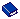POTENTIAL RATE CODE Application Setting

 Group.  OBI Type.  Setting Application. OBI PMS S&C

Description. A Rate Code can be selected to be used to calculate the Potential Rate Amount for one person. The process calculates the selected rate multiplied by physical rooms and this value will be stored in the cube. Dividing potential value by rooms, allows the system to calculate the rate correctly across all levels of the hierarchy. If no Rate Code is selected, the Potential Rate is not populated.

Internal Name. POTENTIAL_RATE_CODE

Property Determination.

 Activation / Inactivation Rules.

 Available Values. Select from the LOV one of the configured rate codes desired for the Potential calculation